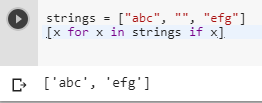2 views
in Python

I want to remove all empty strings from a list of strings in python.

My idea looks like this:

while '' in str_list:

str_list.remove('')

Is there any more pythonic way to do this?

by (106k points)

There are many ways of doing the above problem some are as follows:

• The first thing we can do is we can use filter() method to extract an empty string.

• filter() construct an iterator from those elements of iterable for which function returns true. iterable may be either a sequence, a container that supports iteration, or an iterator. If the function is None, the identity function is assumed, that is, all elements of iterable that are false are removed.

• We can use the following piece of code to that applies filter method:-

str_list = list(filter(None, str_list))

• Another way of solving the above problem is by using List Comprehension.

Below is the code that illustrates the above mentioned method.

strings = ["abc", "", "efg"]

[x for x in strings if x]